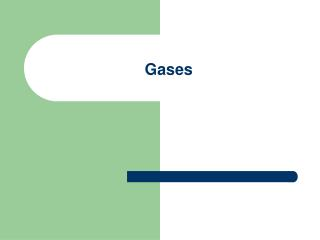Download PresentationGases

# Gases

Download Presentation## Gases

- - - - - - - - - - - - - - - - - - - - - - - - - - - E N D - - - - - - - - - - - - - - - - - - - - - - - - - - -
##### Presentation Transcript

1. Gases

2. Chemistry Review • Atom – smallest piece of an element • Molecule – smallest piece of a compound – two or more atoms chemically bonded • Mole – counting number equal to 6.022 x 1023 • Molar Mass – number of grams of a substance per mole – equal to the atomic mass on the periodic table

3. Review • How many moles in 5.5g of hydrogen gas? • How many molecules in 2.5 moles of oxygen? • What is the mass of one molecule of helium gas?

4. Kinetic Theory • Matter is made of particles in continuous motion • Particles move in random directions at a variety of speeds • Particles of gases are on average very far apart from one another

5. Molecules of gases interact only when they collide • Collisions are perfectly elastic (no loss of kinetic energy)

6. Pressure Units • 1atm = 760mmHg = 760torr = 101kPa • STP – standard temp and pressure (0°C and 1 atm)

7. Gas Laws • Volume is proportional to the temperature – Charles Law ( V1/T1 = V2/T2 ) • Volume is inversely prop to temp – Boyles Law ( P1V1 = P2V2) • Pressure is prop to temp – Gay-Lussac’s Law ( P1/T1 = P2/T2 ) • Combined Gas Law puts them together ( P1V1/T1 = P2V2/T2 )

8. Ideal Gas • One that follows all kinetic theory • Volume of a gas also depends on the number of moles • Ideal Gas Law PV = nRT R = .0821 L•atm/ mol•K

9. Examples • Determine the volume of 1.00 mole of a gas at STP. • A container of oxygen at STP has a volume of 10.0 L. What is the mass of the gas?

10. Examples • A helium party balloon has a radius of 18.0cm at 20°C and 1.05 atm. How many moles of He are in the balloon and what is the mass of the gas in the balloon? • An auto tire is filled to a pressure of 200kPa at 10°C. After driving 100km, the temp rises to 40°C. What is the pressure now?

11. Kinetic Energy of Molecules • The average KE of molecules is directly proportional to the temp KE = ½ mv2 = 3/2 kT k – Boltzman’s Constant = 1.38x10-23J/K

12. Example • What is the average KE of gas molecules at 37°C?

13. Average Velocity • Also called root mean square velocity • vrms = m – mass (in kg) of one molecule of the gas

14. Example • What is the rms speed of an oxygen molecule at 20°C?